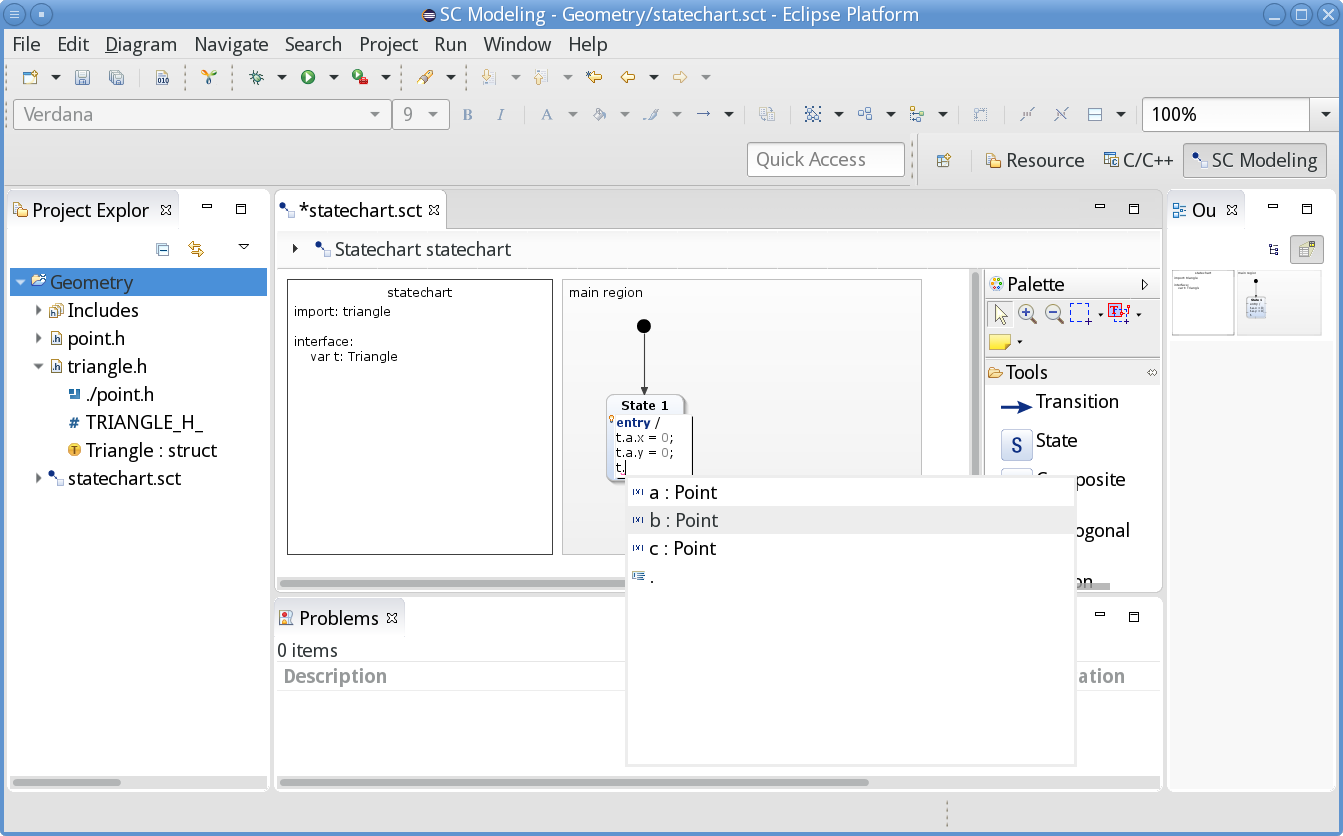## Data structure traversal via dot notation Copy link to clipboard

The dot notation to access structure members can traverse an arbitrary number of stages. As an example, let’s define a datatype named Triangle. A triangle is defined by three points. Using dot notation in a statechart, you can navigate from a triangle to its individual points and further on to the points' coordinates.

The C header file triangle.h specifies the Triangle type:

``````#ifndef TRIANGLE_H_
#define TRIANGLE_H_

#include "./point.h"

typedef struct {
Point a, b, c;
} Triangle;

#endif /* TRIANGLE_H_ */
``````

A Triangle consists of the three Points a, b, and c. Let’s define a Triangle t in a statechart’s definition section as follows:

``````import: "triangle.h"

interface:
var t: Triangle
``````

With this definition we can use expressions like `t.a.x`, see the image below. Regardless of where you are currently editing an expression, you can always use the code assist to explore which fields are available at that very point and of which types these fields are. Example:Content assist in data structure traversal